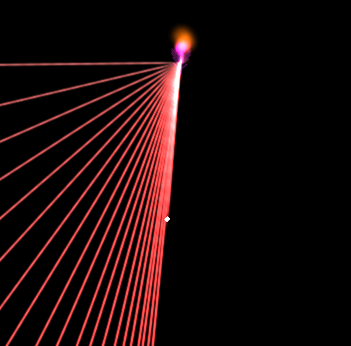# Rotate with constant speed (SOLVED)

#1

I want to make a bot that points rotates to face it’s target. So I went looking for some examples, and found this example.

Relevant code for me being:

``````	local current_rotation = go.get_rotation()
local target_rotation = vmath.quat_rotation_z(target_angle)
local rotation_speed = 4 -- tweak this!
local rotation = vmath.slerp(rotation_speed * dt, current_rotation, target_rotation)
go.set_rotation(rotation)
``````

I noticed that the rotation speed isn’t actually constant here. The vmath.slerp function causes the new rotation to be (in the example) about 6.6% closer to the target rotation than the original rotation. But the closer it gets to the target rotation, the less it will move the next time.

If you excuse this excessive amount of particles being used as lines to demonstrate my point:But I need it to rotate at constant speed. So I need to know how ‘far apart’ the two original quaternions were, how can I do that?

0 Likes

#2

The problem is that you do the calculations of the updated rotation.
If you instead store the initial rotation at the start of the animation, you’ll know where you started, and can keep a constant pace.

Note, be aware that your tweakable constant (if too large) may make the `t` constant exceed `1.0` which is probably not what you want.

Although it’s not possible to use `go.animate()` to animate a quaternion rotation, you can animate the property `euler.z`.

0 Likes

#3

That would work, but my case is similiar to the Britzl’s example; the bot may move, as well as the target. So the target rotation needs to be updated.

For the same reason, I don’t think go.animate() is suitable for the task. I’d need to interrupt previous animation every time the bot or the target moves.

0 Likes

#4

Then, you need to calculate the “rotation left”, i.e to take the difference between the current angle and the target angle, if the step is `> angle_rotation_per_second*dt` then you clamp the rotation to be max `angle_rotation_per_second*dt`.

I think this is easiest done using `euler.z` but is of course possible using quaternions too.

1 Like

#5

After some messing around, this is the solution that appears to be working:

``````local target_rotation = vmath.quat_rotation_z(target_angle)
local angular_distance = math.abs(math.rad(go.get(".", "euler.z")) - target_angle)
local rotation_speed = 4
local clamped_speed = math.min(1, rotation_speed * dt/angular_distance)
local rotation = vmath.slerp(clamped_speed, go.get_rotation(), target_rotation)
go.set_rotation(rotation)
``````

target_angle is in radians in this case.
clamped_speed will be 1 at most, so it’s safe to supply it to vmath.slerp

Thank you for you time Mathias_Westerdahl

3 Likes